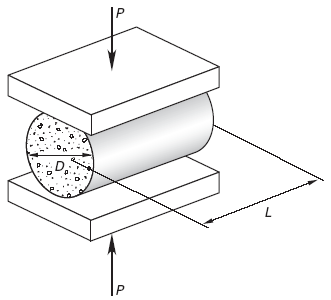Related Resources: calculators

### Concrete Splitting Tensile Strength Test Equations and Calculator

Concrete Splitting Tensile Strength Test Equations and Calculator

The extent and size of cracking in concrete structures are affected to a great extent by the tensile strength of the concrete. The ASTM C496 split cylinder testing procedure is the standard test to determine the tensile strength of concrete. A 6 in"12 in (150 mm"300 mm) cast or drill-core cylinder is placed on its side as in Fig. 1, and the minimum load, P, that causes the cylinder to split in half is used to calculate the splitting tensile strength.Figure 1, Concrete Splitting Tensile Strength Test

ACI 318 Sec. 5.1.4 suggests that the splitting tensile strength fct can be calculated from correlations with compressive strength, and ACI 318 Sec. 8.6.1 indirectly gives such correlations. The general relationship between splitting tensile strength and compressive strength is

Eq. 1, SI Units
fc = 0.56 · λ · f 'c0.5

Eq. 2, U.S.
fc = 6.7 · λ · f 'c0.5

Where:

f 'c = compressive strength of concrete, lbf/in2 (MPa)
λ = Lightweight Aggregate Factors, see table 1
fc = splitting tensile strength, lbf/in2 (MPa)

Lightweight concrete has a lower tensile strength than normalweight concrete, even if both have the same compressive strength. The lightweight aggregate factor, λ, is used to account for this lower tensile strength and is determined from Table 1. For concrete using a blend of lightweight and normalweight aggregates, ACI 318 Sec. 8.6.1 allows for linear interpolation to determine the lightweight aggregate factor. ACI 318 also permits the use of laboratory tests to correlate splitting tensile strength with compressive strength.

Table 1 Lightweight Aggregate Factors, λ

• normal weight concrete = 1.0
• sand-lightweight concrete = 0.85
• all-lightweight concrete = 0.75

Reference

Civil Engineering Reference Manual, Fifteenth Edition, Michael R. Lindeburg, PE

Related